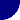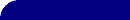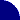# Fitting EIS Data - Diffusion Elements - WarburgRESOURCES > EIS > DIFFUSION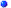Whenever you look at a Complex-Plane Impedance Plot ( Nyquist or Cole-Cole plot) and see a 45° line, or fit data to an equivalent circuit and find a Constant Phase Element (CPE) with an n-value close to 0.5, you should consider diffusion as a possible explanation.MORE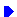##Diffusion Circuit Elements - Warburg

The most common diffusion circuit is the so-called "Warburg" diffusion element, but it is not the only one! A Warburg impedance element can be used to model semi-infinite linear diffusion, that is, unrestricted diffusion to a large planar electrode. This is the simplest diffusion situation because it is only the linear distance from the electrode that matters.

The Warburg impedance is an example of a constant phase element for which the phase angle is a constant 45° and independent of frequency. The magnitude of the Warburg impedance is inversely proportional to the square root of the frequency (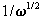) as you would expect for a CPE with an n-value of 0.5. The Warburg is unique among CPE's because the real and imaginary components are equal at all frequencies and both depend upon.

##Warburg Equations

The equation for a Warburg's impedance ( Zw ) is a simple one, but the equation for the Warburg constant (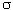) is a bit more complicated and will be discussed on another page.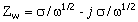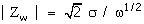A fairly readable derivation of these equations can be found in Bard's book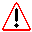We are often suspicious of "diffusion controlled" experiments in aqueous solutions which last more than a few seconds because of the possibilities of convection and stirring at these long times. For exactly the same reason, we should also be suspicious of EIS experiments in aqueous solutions taken at frequencies less than 0.1 Hz if we expect a "diffusion controlled" Warburg impedance!

MOREMore About ... Warburg Impedance PlotsCalculating Diffusion Coefficients Other Diffusion Elements: O, T, GMore About EIS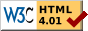© Copyright 2001-2014 Research Solutions & Resources LLC